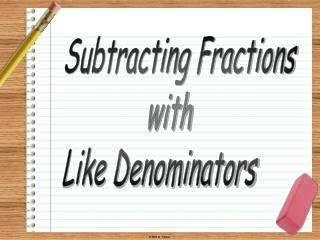DownloadDownload PresentationSubtracting Fractions with Like Denominators

# Subtracting Fractions with Like Denominators

Télécharger la présentation## Subtracting Fractions with Like Denominators

- - - - - - - - - - - - - - - - - - - - - - - - - - - E N D - - - - - - - - - - - - - - - - - - - - - - - - - - -
##### Presentation Transcript

1. Subtracting Fractions with Like Denominators

2. 1 1 1 1 1 1 5 5 5 5 5 5 Subtracting Fractions with Like Denominators -

3. 1 1 1 1 1 1 1 1 1 1 1 1 1 1 1 1 1 1 10 10 10 10 10 10 10 10 10 10 10 10 10 10 10 10 10 10 Subtracting Fractions with Like Denominators - =

4. 1 1 1 1 1 1 1 1 8 8 8 8 8 8 8 8 Subtracting Fractions with Like Denominators -

5. 1 1 1 1 1 1 1 1 7 7 7 7 7 7 7 7 Subtracting Fractions with Like Denominators - =

6. 1 3 Step 2: The denominator remains the same. Step 3: Simplify, if necessary. 1 1 1 Step 1: Subtract the numerators. 15 15 15 1 1 1 1 1 1 1 1 1 1 1 15 15 15 15 15 15 15 15 15 15 15 Subtracting Fractions with Like Denominators 7 15 2 - 15 - 5 5 1 ÷ = 15 5 3 GCF: 5

7. 1 2 Step 2: The denominator remains the same. Step 3: Simplify, if necessary. 1 1 1 1 1 1 1 1 1 1 6 6 6 6 6 6 6 6 6 6 Step 1: Subtract the numerators. Subtracting Fractions with Like Denominators 5 6 2 - 6 - 3 3 1 ÷ = 6 3 2 GCF: 3

8. Step 2: The denominator remains the same. 1 5 Step 3: Simplify, if necessary. 1 1 1 1 1 1 1 1 Step 1: Subtract the numerators. 10 10 10 10 10 10 10 10 Subtracting Fractions with Like Denominators 4 10 2 - 10 - 2 2 1 ÷ = 10 2 5 GCF: 2

9. 1 Step 2: The denominator remains the same. 3 Step 3: Simplify, if necessary. 1 1 1 1 1 1 1 1 9 9 9 9 9 9 9 9 Step 1: Subtract the numerators. Subtracting Fractions with Like Denominators 4 9 1 9 - - 3 3 1 ÷ = 9 3 3 GCF: 3

10. 1 1 1 1 1 1 1 1 1 1 12 12 12 12 12 12 12 12 12 12 1 3 Step 2: The denominator remains the same. Step 3: Simplify, if necessary. Step 1: Subtract the numerators. Subtracting Fractions with Like Denominators 5 12 1 - 12 - 4 4 1 ÷ = 12 4 3 GCF: 4

11. Step 2: The denominator remains the same. Step 3: Simplify, if necessary. 1 1 1 1 1 1 8 8 8 8 8 8 1 4 Step 1: Subtract the numerators. Subtracting Fractions with Like Denominators 3 8 1 - 8 - 2 2 1 ÷ = 8 2 4 GCF: 2

12. Step 2: The denominator remains the same. Step 3: Simplify, if necessary. Step 1: Subtract the numerators. Subtracting Fractions with Like Denominators 5 8 1 8 - 4 1 4 ÷ = 8 4 2 GCF: 4

13. Step 2: The denominator remains the same. Step 3: Simplify, if necessary. Step 1: Subtract the numerators. Subtracting Fractions with Like Denominators 9 11 5 11 - 4 11

14. Step 2: The denominator remains the same. Step 3: Simplify, if necessary. Step 1: Subtract the numerators. Subtracting Fractions with Like Denominators 9 10 5 10 - 2 2 4 ÷ = 10 2 5 GCF: 2

15. Step 2: The denominator remains the same. Step 3: Simplify, if necessary. Step 1: Subtract the numerators. Subtracting Fractions with Like Denominators 9 16 1 16 - 8 1 8 ÷ = 16 8 2 GCF: 8

16. Step 2: The denominator remains the same. Step 3: Simplify, if necessary. Step 1: Subtract the numerators. Subtracting Fractions with Like Denominators 7 12 2 12 - 5 12